## Permutation and Combination: Formulas, Tricks, Examples and Online Test

In mathematics, the notion of permutation is used with several slightly different meanings, all related to the act of permuting (rearranging) objects or values. Informally, a permutation of a set of objects is an arrangement of those objects into a particular order. For example, there are six permutations of the set {1,2,3}, namely (1,2,3) , (1,3,2) , (2,1,3) , (2,3,1) , (3,1,2) , and (3,2,1) . One might define an anagram of a word as a permutation of its letters. The study of permutations in this sense generally belongs to the field of combinatorics.

The number of permutations of n distinct objects is:

n×(n – 1) ×(n – 2) ×… ×2×1, which number is called “n factorial” and written “n!”.

In elementary combinatorics, the name “permutations and combinations” refers to two related problems, both counting possibilities to select k distinct elements from a set of n elements, where for k-permutations the order of selection is taken into account, but for k-combinations it is ignored. However k-permutations do not correspond to permutations as discussed in this article (unless k = n).

### Factorial

A factorial is when a number has an exlamation point after it so it represents all the positive integers leading up to that number then you multiply to solve the factorial.

It is denoted by n!. Therefore, n! = 1 × 2 × 3 × … × (n – 1) × n

For example:

1. 6! = 6 × 5 × 4 × 3 × 2 × 1 = 720
2. 5! = 5 × 4 × 3 × 2 × 1 = 120

#### Formulas

Permutation = nPr = n!/(n-r)!

Combination = nCr = nPr/r!

where, n, r are non negative integers and rn.

• r is the size of each permutation.
• n is the size of the set from which elements are permuted.
• ! is the factorial operator.

Example 1: Find the number of permutations and combinations:
n =6; r = 4.

Solution:

Step 1: Find the factorial of 6.

6! = 6 × 5 × 4 × 3 × 2 × 1 = 720

Step 2: Find the factorial of 6-4.

(6-4) ! = 2! = 2

Step 3: Divide 720 by 2.

Permutation = 720/2 = 360

Step 4: Find the factorial of 4.

4! = 4 × 3 × 2 × 1 = 24

Step 5: Divide 360 by 24.

Combination = 360/24 = 15

### Fundamental Principles of Counting

If there are m ways to do one thing, and n ways to do another, then there are m × n ways of doing both. The Fundamental Counting Principle is the guiding rule for finding the number of ways to accomplish two tasks.

For Examples:

• Let’s say that you want to flip a coin and roll a die. There are 2 ways that you can flip a coin and 6 ways that you can roll a die. There are then 2 × 6 = 12 ways that you can flip a coin and roll a die.
• If you want to hit one note on a piano and play one string on a banjo, then there are 88 × 5 = 440 ways to do both.
• If you want to draw 2 cards from a standard deck of 52 cards without replacing them, then there are 52 ways to draw the first and 51 ways to draw the second, so there are a total of 52 × 51 = 2652 ways to draw the two cards.

If an event can occur in ‘m’ ways and another event can occur in ‘n’ ways independent of the first event, then either of the two events can occur in (m + n) ways.

Example 2: In a class there are 10 boys and 8 girls. The class teacher wants to select a student for monitor of the class. In how many ways the class teacher can make this selection?

Solution: The teacher can select a student for monitor in two exclusive ways

1. Select a boy among 10 boys, which can be done in 10 ways OR
2. Select a girl among 8 girls, which can be done in 8 ways.

Hence, by the fundamental principle of addition, either a boy or a girl can be selected in 10 + 8 = 18 ways.

### Principle of Multiplication

If an operation can be performed in ‘m’ ways and after it has been performed in any one of these ways, a second operation can be performed in ‘n’ ways, then the two operations in succession can be performed in (m × n) ways.

Example 3: In a class there are 10 boys and 8 girls. The teacher wants to select a boy and a girl to represent the class in a function. In how many ways can the teacher make this selection?

Solution: The teacher has to perform two jobs :

1. To select a boy among 10 boys, which can be done in 10 ways.
2. To select a girl, among 8 girls, which can be done in 8 ways.

Hence, the required number of ways = 10 × 8 = 80.

### Methods of Sampling

1. Sampling process can be divided into following forms :
2. The order is IMPORTANT and the repetition is ALLOWED, each sample is then a SEQUENCE.
3. The order is IMPORTANT and the repetition is NOT ALLOWED, each sample is then a PERMUTATION.
4. The order is NOT IMPORTANT and repetition is ALLOWED, each sample is then a MULTISET.
5. The order is NOT IMPORTANT and repetition is NOT ALLOWED, each sample is then a COMBINATION.

## Permutation

A permutation is an arrangement of objects, without repetition, and order being important. Another definition of permutation is the number of such arrangements that are possible.

The number of permutations of ‘n’ things taken ‘r’ at a time is denoted by nPr It is defined as, nPr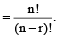Since a permutation is the number of ways you can arrange objects, it will always be a whole number. The denominator in the formula will always divide evenly into the numerator.

This also gives us another definition of permutations. nPn = n!

Example 4: List all permutations of the letters ABCD

Solution:

Now, if you didn’t actually need a listing of all the permutations, you could use the formula for the number of permutations. There are 4 objects and you’re taking 4 at a time.

4P4 = 4!/(4 – 4) ! = 4!/0! = 24/1 = 24.

Example 5: List all three letter permutations of the letters in the word HAND

Solution:

 HAN HNA HAD HDA HND HDN AHN ANH AHD ADH AND ADN NHD NDH NAH NHA NAD NDA DHA DAH DAN DNA DHN DNH

Now, if you didn’t actually need a listing of all the permutations, you could use the formula for the number of permutations. There are 4 objects and you’re taking 3 at a time.

P³ = 4!/(4-3) ! = 4!/1! = 24/1 = 24.

### Cyclic Permutations

It is a permutation around a circular object, it must be noted, a circular object has no beginning and no end, we fix one object (or person) and permute the remaining.

Arrangements round a circular table

Consider five persons A, B, C, D and E to be seated on the circumference of a circular table in order (which has no head) . Now, shifting A, B, C, D and E one position in anticlockwise direction we will get arrangements as follows: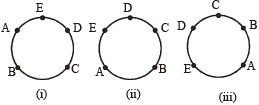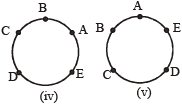we see that arrangements in all figures are same.

∴ The number of circular permutations of n different things taken all at a time is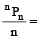(n – 1) !, if clockwise and anticlockwise orders are taken as different.

Example 6: In how many ways can 6 students of a school be seated around a circular table?

Solution: The problem is a cyclic permutation

The number of ways the 6 students can be seated

= 1 × (6 – 1) ! = 5! = 5 × 4 × 3 × 2 × 1 = 120

#### Arrangements of beads or flowers (all different) around a circular necklace or garland

Consider five beads A, B, C, D and E in a necklace or five flowers A, B, C and D, E in a garland etc. If the necklace or garland on the left is turned over we obtain the arrangement on the right, i.e., anticlockwise and clockwise order of arrangements are not different.

Thus the number of circular permutations of ‘n’ different things taken.all at a time is:

1/2 (n–1)!, if clockwise and anticlockwise orders are taken to be some.

Example 7: The number of ways in which 10 persons can sit round a circular table so that none of them has the same neighbours in any two arrangements.

Solution: 10 persons can sit round a circular table in 9! ways. But here clockwise and anticlockwise orders will give the same neighbours. Hence the required number of ways = 1/2 × 9!

### Conditional Permutations

When conditions are applied to the way things arranged, it then happen that permutation is said to be conditional.

Number of permutations of n things taking r at a time, in which a particular thing always occurs =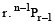.

Example 8: How many 4 digits number (repetition is not allowed) can be made by using digits 1-7 if 4 will always be there in the number?

Solution: Total digits (n) = 7

Total ways of making the number if 4 is always there

= r × n-1Pr-1 = 4 × ⁶P³ = 480.

### Distinguishable Permutations

Suppose a set of n objects has n₁ of one kind of object, n₂ of a second kind, n₃ of a third kind, and so on, with n = n₁ + n₂ + n₃ + . . . + nk, Then the number of distinguishable permutations of the n objects is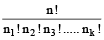Example 9: In how many distinguishable ways can the letters in BANANA be written?

Solution: This word has six letters, of which three are A’s, two are N’s, and one is a B. Thus, the number of distinguishable ways the letters can be written is: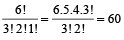Number of permutations of n things taking r at a time, in which a particular thing never occurs =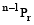.

Example 10: How many different 3 letter words can be made by 5 vowels, if vowel ‘A’ will never be included?

Solution: Total letters (n) = 5

So total number of ways = n-1Pr = 5-1P3 = 4P3 = 24.

Number of permutations of n different things taking all at a time, in which m specified things always come together = m!(n-m+1).

Example 11: In how many ways can we arrange the five vowels, a, e, i, o and u if:

1. two of the vowels e and i are always together.
2. two of the vowels e and i are never together.

Solution: 1. Using the formula m!(n – m + 1)!

Here n = 5, m = 2(e and i)

⇒ Required no. of ways = 2!(5 – 2 + 1) ! = 2 × 4! = 48

2. No. of ways when e & i are never together

= total no. of ways of arranging the 5 vowels

– no. of ways when e & i are together = 5! – 48 = 72

Or use n! – m!(n – m + 1) ! = 5! – 48 = 72

Number of permutations of n different things taking all at a time, in which m specified things never come together = n!-m!(n-m+1)!

The number of permutations of ‘n’ things taken all at a time, when ‘p’ are alike of one kind, ‘q’ are alike of second, ‘r’ alike of third, and so on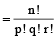.

Example 12: How many different words can be formed with the letters of the world MISSISSIPPI.

Solution: In the word MISSISSIPPI, there are 4 I’s, 4S’s and 2P’s.

Thus required number of words =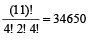The number of permutations of ‘n’ different things, taking ‘r’ at a time, when each thing can be repeated ‘r’ times = nr

Example 13: In how many ways can 5 prizes be given away to 4 boys, when each boy is eligible for all the prizes?

Solution: Any one of the prizes can be given in 4 ways; then any one of the remaining 4 prizes can be given again in 4 ways, since it may even be obtained by the boy who has already received a prize.

Hence 5 prizes can be given 4 × 4 × 4 × 4 × 4 = 4⁵ ways.

## Combination

A combination is an arrangement of objects, without repetition, and order not being important. Another definition of combination is the number of such arrangements that are possible.

The number of combinations of ‘n’ dissimilar things taken ‘r’ at a time is denoted by nCr or C(n, r) . It is defined as, nCr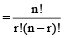The key points to a combination are that there is no repetition of objects allowed and the order isn’t important.

Example 14: List all combinations of the letters ABCD in groups of 3.

Solution: There are only four combinations (ABC, ABD, ACD, and BCD) . Listed below each of those combinations are the six permutations that are equivalent as combinations.

 ABC ABD ACD BCD ABC ABD ACD BCD ACB ADB ADC BDC BAC BAD CAD CBD BCA BDA CDA CDB CAB DAB DAC DBC CBA DBA DCA DCB

Example 15: In how many ways a hockey team of eleven can be elected from 16 players?

Solution: Total number of ways

=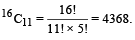=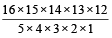= 4368.

### Conditional Combinations

Number of combinations of n distinct things taking r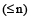at a time, when k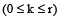particular objects always occur =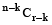.

Number of combinations of n distinct objects taking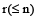at a time, when kparticular objects never occur =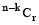Number of selections of r things from n things when p particular things are not together in any selection = nCrn-pCr-p

Number of selection of r consecutive things out of n things in a row = n – r + 1

Number of selection of r consecutive things out of n things along a circle=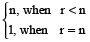The number of Combinations of ‘n’ different things taking some or all at a time =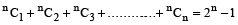The number of ways of dividing ‘m + n’ things into two groups containing ‘m’ and ‘n’ things respectively = m+nCn . nCn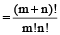The number of ways of dividing ‘m + n + p’ things into three groups containing ‘m’, ‘n’ and ‘p’ things respectively = m+n+pCm.n+pCp=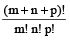(i) If m = n = p ie. ‘3m’ things are divided into three equal groups then the number of combinations is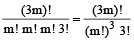(ii) Buf if ‘3m’ things are to be divided among three persons, then the number of divisions is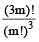If mn distinct objects are to be divided into m groups. Then, the number of combination is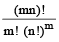, when the order of groups is not important

and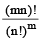, when the order of groups is important

### Number of Rectangles and Squares

Number of rectangles of any size in a square of size n × n is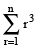and number of squares of any size is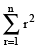.

Number of rectangles of any size in a rectangle size n × p (n < p) is (np/4) (n + 1) (p + 1) and number of squares of any size is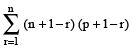.

Example 16: How many squares can be formed on a chessboard?

Solution: A chessboard is made up of 9 equispaced horizontal and vertical line. To make a 1 × 1 square, we must choose two consecutive horizontal and vertical lines from among these. This can be done in 8 × 8 = 8² ways.

A 2 × 2 square needs three consecutive horizontal and vertical lines, and we can do this in 7 × 7 = 7² ways. Continuing in this manner, the total number of square is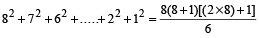= 204.

### Points to Remember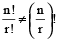0! = 1

The factorials of fractions and negative integers are not defined.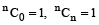;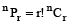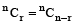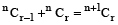nCx = nCy ⇒ x + y = n

nCr = nCr+1= n+1Cr

nCr =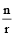. n-1Cr-1

nCr =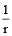(n – r + 1) nCr-1

nC1 = nCn-1 = n

## Solved Examples

1.Prove that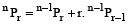Solution: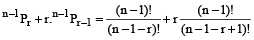=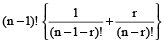=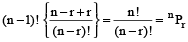2. Prove that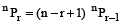Solution: We have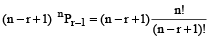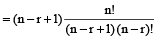=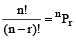3. There are 6 multiple choice questions in an examination. How many sequences of answers are possible, if the first three questions have 4 choices each and the next three have 5 choices each?

Solution: Each of the first three questions can be answered in 4 ways and each of the next three questions can be answered in 5 different ways.

Hence, the required number of different sequences of answers

= 4 × 4 × 4 × 5 × 5 × 5 = 8000.

4. Five persons entered a lift cabin on the ground floor of an 8-floor house. Suppose that each of them can leave the cabin independently at any floor beginning with the first. What is the total number of ways in which each of the five persons can leave the cabin at any of the 7 floors?

Solution: Any one of the 5 persons can leave the cabin in 7 ways independent of other.

Hence the required number of ways = 7 × 7 × 7 × 7 × 7 = 7⁵.

5. In how many different ways can five boys and five girls form a circle such that the boys and girls are alternate?

Solution: After fixing up one boy on the table the remaining can be arranged in 4! ways.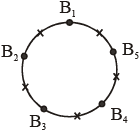There will be 5 places, one place each between two boys

which can be filled by 5 girls in 5! ways.

Hence by the principle of multiplication, the required number of ways = 4! × 5! = 2880.

6. In how many ways can 5 boys and 5 girls be seated at a round table no two girls may be together?

Solution: Leaving one seat vacant between two boys may be seated in 4! ways. Then at remaining 5 seats, 5 girls any sit in 5! ways. Hence the required number = 4! × 5!

7. How many numbers of 3 digits can be formed with the digits 1, 2, 3, 4, 5 when digits may be repeated?

Solution: The unit place can be filled in 5 ways and since the repetitions of digits are allowed, therefore, tenth place can be filled in 5 ways.

Furthermore, the hundredth place can be filled in 5 ways also.

Therefore, required number of three digit numbers is 5 × 5 × 5 = 125.

8. In how many ways 8 persons can be arranged in a circle?

Solution: The eight persons can be arranged in a circle in

(8 – 1) ! = 7! = 5040.